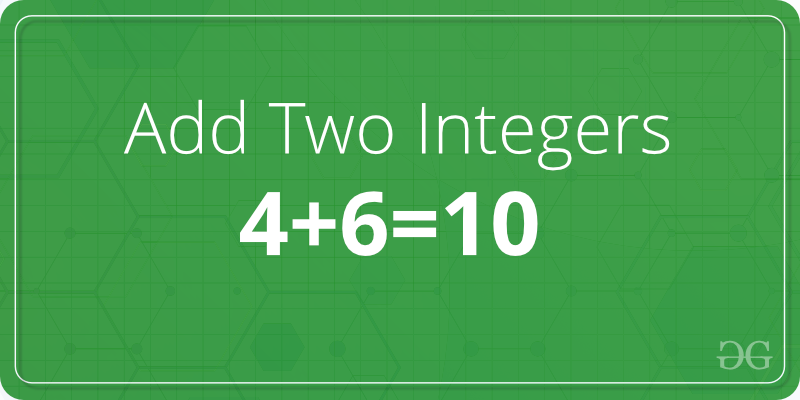# C Program to Add two Integers

Given two numbers A and B. The task is to write a program to find the addition of these two numbers.Examples:

```Input: A = 2, B = 3
Output: 5

Input: A = 3, B = 6
Output: 9
```

## Recommended: Please try your approach on {IDE} first, before moving on to the solution.

In the below program to add two numbers, the user is first asked to enter two numbers and the input is scanned using the scanf() function and stored in the variablesand. Then, the variablesandare added using the arithmetic operatorand the result is stored in the variable sum.

Below is the C program to add two numbers:

 `// C program to add two numbers ` `#include ` ` `  `int` `main() ` `{ ` `    ``int` `A, B, sum = 0; ` `     `  `    ``// Ask user to enter the two numbers ` `    ``printf``(``"Enter two numbers A and B : \n"``); ` `     `  `    ``// Read two numbers from the user || A = 2, B = 3 ` `    ``scanf``(``"%d%d"``, &A, &B); ` `     `  `    ``// Calclulate the addition of A and B ` `    ``// using '+' operator ` `    ``sum = A + B; ` `     `  `    ``// Print the sum ` `    ``printf``(``"Sum of A and B is: %d"``, sum); ` `     `  `    ``return` `0; ` `} `

Output:

```Enter two numbers A and B : 2 3
Sum of A and B is: 5
```

My Personal Notes arrow_drop_upCheck out this Author's contributed articles.

If you like GeeksforGeeks and would like to contribute, you can also write an article using contribute.geeksforgeeks.org or mail your article to contribute@geeksforgeeks.org. See your article appearing on the GeeksforGeeks main page and help other Geeks.

Please Improve this article if you find anything incorrect by clicking on the "Improve Article" button below.

Article Tags :

2

Please write to us at contribute@geeksforgeeks.org to report any issue with the above content.